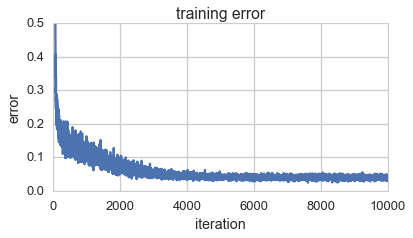# Watch Tiny Neural Nets Learn

In this post I'll show you some animations of tiny neural nets learning. Finding the right neural net for a given problem needs experience and experimentation. I'll show you the steps and missteps it took me to find a good net to predict a noisy sine function.

I'll assume you already know the very basics of neural nets in Keras, which you can read up on in my post First Steps With Neural Nets in Keras.

This is the training data for the tiny nets. It's a sine plus some normally distributed error.

In :
expand code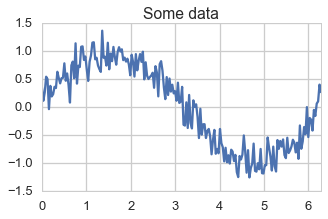Let's first try a net with 1 layer of 60 neurons. The model has 120 parameters. The 60 weights from the input to the 60 neurons and the 60 weights form the neurons to the output.

In :
from keras.models import Sequential
from keras.layers.core import Dense, Activation

n_conn = 60
model = Sequential()
model.compile(loss='mean_squared_error', optimizer='sgd')

In :
expand code
In :
history = TrainingHistory()
res = model.fit(X_train,
Y_train,
nb_epoch=5000,
verbose=0,
callbacks=[history])

In :
expand code
In :
visualize_training(history, 'tiny-sine-one-layer')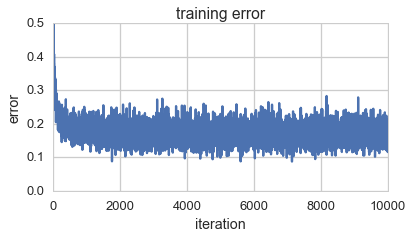This is how the predictions of the net look during each training iteration.

The net quickly learns the first hill of the sine function. This corresponds to the training error decreasing rapidly during the first few iterations. Then it gets stuck in a local optimum. The training error remains in the range fo 0.2 without any signs of improvement over the last 17000 iterations.

Let's try another configuration of the net. Let's have two layers of 10 neurons each. This net has again 120 parameters. The 10 weights from the input to the first 10 neurons, the weights for the 100 connections from the first 10 to the second 10 neurons and the 10 from the second neurons to the output.

In :
n_conn = 10
model = Sequential()
model.compile(loss='mean_squared_error', optimizer='sgd')

X_train = np.array(x, ndmin=2).T
Y_train = np.array(y, ndmin=2).T
history = TrainingHistory()
model.fit(X_train,
Y_train,
nb_epoch=5000,
verbose=0,
callbacks=[history])

visualize_training(history, 'tiny-sine-two-layer')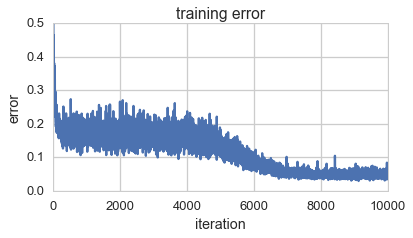This looks better. But the way the training error improved looks like it could have gotten stuck again. As it looks like a problem of a local optimum, let's try using a different optimizer. A reommended optimizer is Adam. You can find a readable explanation and the recommendation in this Stanford lecture.

In :
from keras.optimizers import Adam

n_conn = 60
model = Sequential()

X_train = np.array(x, ndmin=2).T
Y_train = np.array(y, ndmin=2).T
history = TrainingHistory()
model.fit(X_train,
Y_train,
nb_epoch=5000,
verbose=0,
callbacks=[history])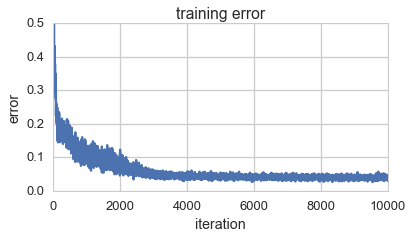This time we see steady decrease in the training error. And the final prediction looks close to optimal. A 2-layer network worked better before, let's see how the 2 layers work with the new optimizer.

In :
n_conn = 10
model = Sequential()

X_train = np.array(x, ndmin=2).T
Y_train = np.array(y, ndmin=2).T
history = TrainingHistory()
model.fit(X_train,
Y_train,
nb_epoch=5000,
verbose=0,
callbacks=[history])